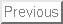Next: $B;29MJ88%(B $BHs@~7A(B Schrodinger $B7?J}Dx<0$N:nMQAGO@E*07(B

$B2,Bt(B $BEP!JEl5~M}2JBg3X!&M}3XIt!K(B

The purpose of this talk is to present a new existence theorem for the nonlinear Schrodinger equation. The theorem is an answer to the open conjecture.

Let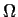be a bounded or unbounded domain in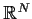with compact C2-boundary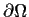. In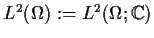we consider the nonlinear Schrodinger equation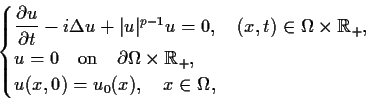(NLS)

where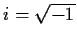, the exponent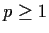is a constant and u is a complex-valued unknown function. It is known that (NLS) admits a unique global strong solution under the following condition:
•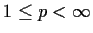if N=1,2,
•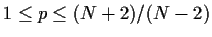if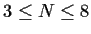(Pecher and von Wahl , 1979),
•if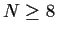(Shigeta , 1986).

The following conjecture remains open.

Conjecture (Pecher and von Wahl , 1979). p0:=(N+2)/(N-2) is the largest possible exponent for the global existence of strong solutions to (NLS).

However, we can prove the global existence for all exponentsbeyond their conjecture.

Theorem (). Let. Then for any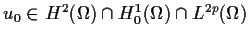there exists a unique global strong solution u(t):=u(x,t) to (NLS) in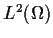such that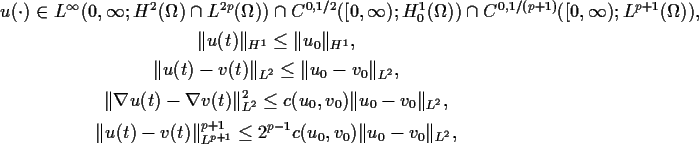where
v(t) is a solution to (NLS) with initial value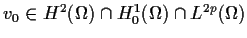.Next: $B;29MJ88%(B Tohru Okuzono $BJ?@.(B12$BG/(B6$B7n(B5\$BF|(B